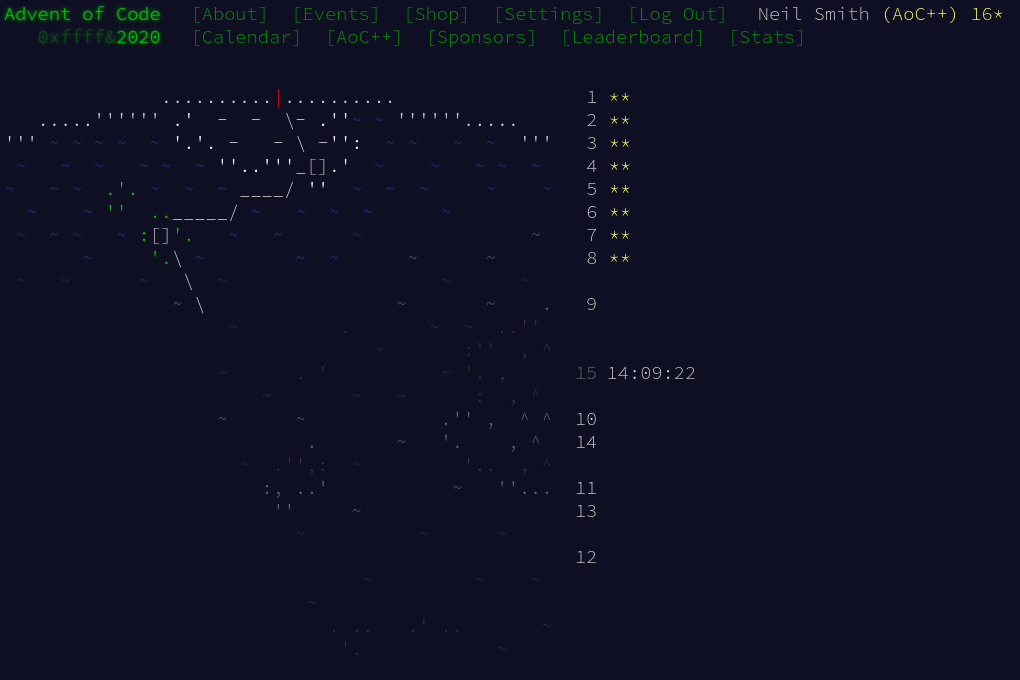December 14, 2020

# Advent of Code 2020 day 8

Return of the VM!It had to happen sometime! Day 8 was the first virtual machine to simulate. First off,  a couple of data structures for storing the machine (the need for the MachineResult only became apparent in part 2).

data Instruction = Acc Int | Jmp Int | Nop Int
deriving (Show, Eq)

type Program = V.Vector Instruction

data Machine = Machine
{ machineProgram :: Program
, machineIP :: Int
, machineAcc :: Int
}

data MachineResult = Looped Int | Terminated Int | OutOfBounds Int Int
deriving (Show, Eq)


The fields in the Machine record are the program, the instruction pointer (location of the next instruction to execute) and the current value of the accumulator.

Reading in the machine was simple, just reading the text and creating a program. The interesting thing was I decided to use a Vector for the program, rather than a List.

instructionP = accP <|> jmpP <|> nopP

accP = Acc <$> ("acc " *> signed decimal) jmpP = Jmp <$> ("jmp " *> signed decimal)
nopP = Nop <$> ("nop " *> signed decimal) programP = V.fromList <$> sepBy instructionP endOfLine


## Part 1

For executing the machine, I kept a separate Set of visited IPs and executed the machine one step at a time. (I did think about using some form of State or Reader monad for the machine, but decided that it wasn't worth the effort for something so simple.)

part1 machine = executeMany S.empty machine

executeMany visited machine
| currentIP S.member visited = Looped (machineAcc machine)
| currentIP == programSize     = Terminated (machineAcc machine)
| currentIP > programSize      = OutOfBounds (machineAcc machine) currentIP
| otherwise                    = executeMany visited' machine'
where machine' = executeStep machine
currentIP = machineIP machine
visited' = S.insert currentIP visited
programSize = V.length $machineProgram machine executeStep m = execute ((machineProgram m)!(machineIP m)) m execute (Acc n) m = m { machineIP = machineIP m + 1 , machineAcc = machineAcc m + n } execute (Jmp n) m = m { machineIP = machineIP m + n } execute (Nop n) m = m { machineIP = machineIP m + 1 }  ## Part 2 Brute force was the easiest way to go for this. I mutated the program by replacing, one at at time, the nop and jmp instructions; then I ran all the mutated programs to see which reached the "termination" condition. part2 program = filter terminates$ map runProgram programs
where programs = altPrograms program
runProgram p = executeMany S.empty (Machine p 0 0)
terminates (Terminated _) = True
terminates (OutOfBounds _ _) = True
terminates _ = False

altPrograms program = map (mutateProgram program) [0..(V.length program - 1)]

mutateProgram program i = go (program!i)
where go (Nop n) = program // [(i, Jmp n)]
go (Jmp n) = program // [(i, Nop n)]
go _ = program


As promised, there was only one variant program that Terminated, and none that failed with an OutOfBounds condition.

## Code

You can find the code here or on Gitlab.